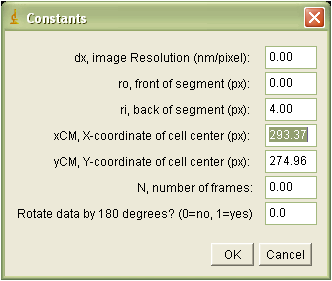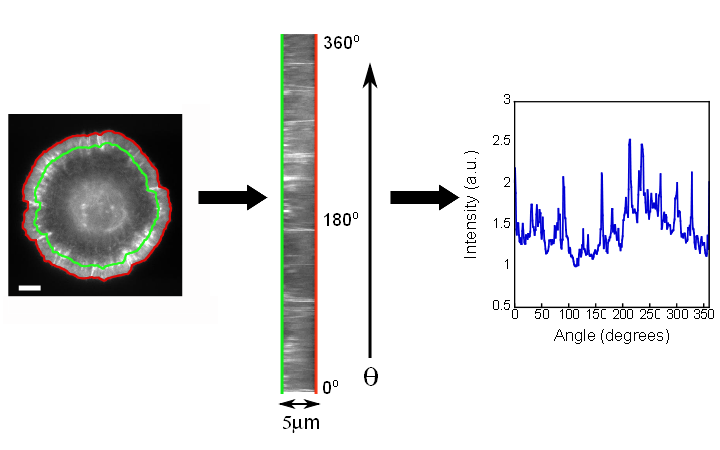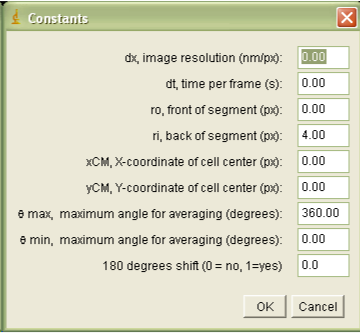# Leading Edge Intensity  and Calculations

 JFilament Speckle TrackerJ Leading Edge Position and Velocity Leading Edge Intensity Correlation Analysis Speckle Velocities

Total Intensity:

The plugin Intensity_Ribbon calculates the total intensity in a "ribbon" of user-defined
width along the arc length of the leading edge for a selected image stack. Like LE_Velocity it requires a snake file generated in JFilament to indicate the position of the cell's leading edge. Also, like LE_Velocity, it maps contours to polar coordinates. When  Intensity_Ribbon is run it will prompt the user to input necessary data:The required parameters are:

• dx: image resolution, measured in nm/pixel.
• ro: the distance from the leading edge to the outer boundary of the lamellipodia section to be measured (negative values are outside the cell)
• ri: the distance from the leading edge to the inner boundary of the lamellipodia section to be measured (negative values are outside the cell)
•  xCM: the x-coordinate of the cell center, measured in pixels
• yCM: the y-coordinate of the cell center, measured in pixels
• N: number of frames in the stack
• Rotation, which allows the user to rotate the angles of the ouput by 180 degrees. (Useful for sections of cells.)
The user is then prompted to choose a snake file.

Outputs:

Data file: The file contains a matrix of intensities of dimentions N x 360. The x-coordinate of this matrix indicates the frame number, while the y coordinate of this matrix indicates angle. The total intensity is measured in intensity units of original image. The plugin does not subtract background intensity.

Image stack:
The generated stack of 'ribbons' of lamellipodia displays Intensity as a function of angle and distance from leading edge, for all time frames. Below, an example is shown where the outer and inner boundaries of the  measuring 'ribbon' of lamellipodium are drawn in red and green, respectively.Intensity_Profile:

The Intensity_Profile plugin calculates the intensity as a function of distance from the leading edge
for a selected image stack. Like Intensity_Ribbon it requires a snake file generated in JFilament to indicate the position of the cell's leading edge, and maps contours to polar coordinates. When  Intensity_Profile is run it will prompt the user to input necessary data:The required parameters are:
• dx: image resolution, measured in nm/pixel.
• dt: time per frame, measured in s.
• ro: the distance from the leading edge to the outer boundary of the lamellipodia section to be measured (negative values are outside the cell)
• ri: the distance from the leading edge to the inner boundary of the lamellipodia section to be measured (negative values are outside the cell)
•  xCM: the x-coordinate of the cell center, measured in pixels.
• yCM: the y-coordinate of the cell center, measured in pixels.
• qmax: the maximum angle over which to average data, in degrees
• qmin: the minimum angle over which to average data, in degrees
• Rotation, which allows the user to rotate the angles of the ouput by 180 degrees. (Useful for sections of cells.)
Once the user inputs these data, Intensity_Profile will prompt the user to choose a snake file.

Outputs:

Data file: The file containing intensity measurements as functions of time, angle, and distance from the leading edge. The data is formatted in columns that provide: time(s), angle (degrees), distance from the leading edge (
µm), and intensity. The plugin does not subtract background intensity.

Image Stack: The intensity of each pixel is the average intensity calculated over the provided range of angles.
The y-position of each image in the stack indicates the distance from the leading edge, and each frame is a different time.

References:

• G. L. Ryan, N. Watanabe, D. Vavylonis, "Image Analysis Tools to Quantify Cell Shape and Protein Dynamics near the Leading Edge," Cell Structure and Function (2013).
• G. L. Ryan, H. Petroccia, N. Watanabe, D. Vavylonis, "Excitable Actin Dynamics in Lamellipodial Protrusion and Retraction," Biophys. J. 102:1493-1502 (2012).